# BJT Common Emitter Large and Small Signal Research

#### cikalekli

Joined Dec 10, 2020
103
Hi, I want to study about BJT Common Emitter Large (or bias) and Small Amplifier Circuits.

My question is I could not find a decent sufficient resource neither from youtube nor from google.I want to study about those common emitter large & small signal models, analysis, ac gain, input & output impedance.

Please, is there any resource you can recommend me?

After studying, I want to become knowledgeable about turning the BJT circuit into lage and small model as well...

Here is an example that wanted from us to find its small & large signal model, Ic and also Vout:
(This is just for an example)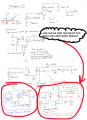I really have to study about those topics in this week but my teacher did not give me some sufficient sources to understand them.

If you help me out for finding sources, I will be able to study a lot and that will make me such a happy person. ^^

#### SamR

Joined Mar 19, 2019
4,548
•cikalekli

#### LvW

Joined Jun 13, 2013
1,622
In addition, here is a short remark regarding the small-signal model you have shown:
In the right part of the model there is a voltage-controlled current source ic=gm*vbe.
From the physics point of view, this is a "correct" model (reflecting the real behaviour of the BJT).
However, you should not be confused if you see another model with a current-controlled current source ic=beta*ib.
This model can also be used for calculations because of beta=gm*rbe.

•atferrari and cikalekli

#### cikalekli

Joined Dec 10, 2020
103
In addition, here is a short remark regarding the small-signal model you have shown:
In the right part of the model there is a voltage-controlled current source ic=gm*vbe.
From the physics point of view, this is a "correct" model (reflecting the real behaviour of the BJT).
However, you should not be confused if you see another model with a current-controlled current source ic=beta*ib.
This model can also be used for calculations because of beta=gm*rbe.
Thank you so much for this additional vital information.

I always got stuck whenever I try to detect which small signal model should I use in the questions...

If there is a source which explains how many different types can be as a small signal model, could you please share with me as well?

I mean I would really appreciate if this is possible

#### Bordodynov

Joined May 20, 2015
3,037
•Jony130

#### LvW

Joined Jun 13, 2013
1,622

I always got stuck whenever I try to detect which small signal model should I use in the questions...
At first, I would recommend not to consider the small-signal models as a very important help. These models are nothing else than a visual interpretations of the basic equations describing the BJT behaviour.
More than that, you should handle and use these models "with care".
For example, because one of the models shows the signal collector current as a current-controlled current source (ic=beta*ib) many people believe (and, unfortunately, also some books and other sources) that the BJT would be a current-controlled device.
But this is wrong and in contradiction to all observable effects and properties of real BJT-based applications (typical example: Current mirror).
We all kow that the voltage gain of a gain stage is independent on the beta values and only determined by the DC collector current (and the coresponding transconductance gm).
As to your last question: There is a third model (T-model) which I personally would NEVER recommend (although it "works"). In this model the inverse transconductance gm=beta/rbe is used as a so-called "intrinsic emitter resistance" (re=1/gm). This is - for my opinion - a very misleading and confusing approach. The expression rbe/gm is, of course, not a property of the emitter but connects input and output paremeters with each other.
So I am afraid that such a view would prevent the novice from developing a realistic and correct idea of how the transistor works.

Last edited:
•cikalekli

#### LvW

Joined Jun 13, 2013
1,622
@Bordodynov, may I ask you for some explanations to your simulations? What is the reason to use fixed (and stepped) current sources for Ic and Ie, respectively? To me, this seems to be not very close to reality. When you intend to simulate the ratio Ic/Ib for different currents, why not use an approach that is close to reality ? I would recommend to simulate Ic=f(Vbe) displaying the desired current ratio .

#### cikalekli

Joined Dec 10, 2020
103
At first, I would recommend not to consider the small-signal models as a very important help. These models are nothing else than a visual interpretations of the basic equations describing the BJT behaviour.
More than that, you should handle and use these models "with care".
For example, because one of the models shows the signal collector current as a current-controlled current source (ic=beta*ib) many people believe (and, unfortunately, also some books and other sources) that the BJT would be a current-controlled device.
But this is wrong and in contradiction to all observable effects and properties of real BJT-based applications (typical example: Current mirror).
We all kow that the voltage gain of a gain stage is independent on the beta values and only determined by the DC collector current (and the coresponding transconductance gm).
As to your last question: There is a third model (T-model) which I personally would NEVER recommend (although it "works"). In this model the inverse transconductance gm=beta/rbe is used as a so-called "intrinsic emitter resistance" (re=1/gm). This is - for my opinion - a very misleading and confusing approach. The expression rbe/gm is, of course, not a property of the emitter but connects input and output paremeters with each other.
So I am afraid that such a view would prevent the novice from developing a realistic and correct idea of how the transistor works.
Ah Thank you for your detailed explanation.
I will pay attention to the important details you mentioned...

#### cikalekli

Joined Dec 10, 2020
103
Thank you so much with that simulation view proof.
Now it seems to me more clearer than before. ^^

#### Bordodynov

Joined May 20, 2015
3,037
@Bordodynov, may I ask you for some explanations to your simulations? What is the reason to use fixed (and stepped) current sources for Ic and Ie, respectively? To me, this seems to be not very close to reality. When you intend to simulate the ratio Ic/Ib for different currents, why not use an approach that is close to reality ? I would recommend to simulate Ic=f(Vbe) displaying the desired current ratio .
LvW
You have an obsession with proving that the bipolar transistor is driven by current. This is akin to the chicken and egg problem. I prefer to think of it as current controlled. For some reason, transistor manufacturers give the current gain as a function of current. From this it is more convenient to stick to the concept of current control. When I soldered my first transistor circuit, I measured the current gain and then calculated the base resistor and my circuit had the DC mode that I wanted. And how in this case did you calculate the ratings in the collector and base circuits using the concept of voltage control. I am not claiming that you are wrong. To me, the theory that makes circuit design easier is acceptable. Any element has a volt-ampere characteristic. You can plot the voltage vs. current, or you can plot the current vs. voltage. Let's take the simpler case of an LED. What do you think controls it. If you assume voltage, there could be a problem. If it is a high-power LED, there may be a problem with powering it with a voltage source. It can burn out. Temperature changes can change the current and the power dissipation.
I. do not understand the question about discrete current values. Always when taking characteristics and graphs use fixed values of the argument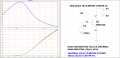Always when taking characteristics and graphs use fixed values of the argument. And what is far from the practice? Maybe you just do not understand what I do. And I am doing with the help of controlled sources the desired collector-emitter voltage. It's easier for me to do it in a simulator. I moved the current source from the collector circuit to the emitter circuit. And I built the current amplification from the base-emitter voltage. It turned out to be a little more difficult to do. I had to calculate the limits of the base-emitter voltage. Here's another example of the wretchedness of your approach.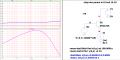#### LvW

Joined Jun 13, 2013
1,622
LvW
You have an obsession with proving that the bipolar transistor is driven by current. This is akin to the chicken and egg problem. I prefer to think of it as current controlled. For some reason, transistor manufacturers give the current gain as a function of current. From this it is more convenient to stick to the concept of current control. When I soldered my first transistor circuit, I measured the current gain and then calculated the base resistor and my circuit had the DC mode that I wanted. And how in this case did you calculate the ratings in the collector and base circuits using the concept of voltage control. I am not claiming that you are wrong. To me, the theory that makes circuit design easier is acceptable. Any element has a volt-ampere characteristic. You can plot the voltage vs. current, or you can plot the current vs. voltage.
I am bit surprised that you consider my position as an "obsession".
Is it really necessary to give you references (books, university papers,...)?
I think, it should be possible to have a technical discussion without such polemic remarks.
Of course, you are free to "think of it as a current-controlled" device.
But the fact is that - physically spoken - the BJT is voltage controlled. This can be prooved.
As I have mentioned - all (I repeat: all) observations and explanations show this. Do you have any counter example?
There is not a single proof for current-control! It is just a claim caused by a misinterpretation of the equation Ic=B*Ib.

By the way: In physics we never have a "chicken-egg-problem". A current is always the result of a driving voltage; in reality, there are no current sources. To speak about "current injection" is a simplified kind of "labor jargon".

But I think, a good engineer should be able to discriminate between (a) physical facts and (b) formulas and design rules.
Of course, all manufacturers specify the beta-value because they are important parameters which deterrmine the input resistance.

Finally, may I kindly ask you to mention one single application where the BJT is (and must be) considered as a current-controlled device? That would certainly be a great help to be able to continue the discussion on a more factual-technical level.

Last edited:

#### Bordodynov

Joined May 20, 2015
3,037
I don't need any references. I use a concept that allows me to simply calculate circuits and use reference data. Instead you suggest I use exponential functions. There is one nuance. There is always a parasitic base resistance in a transistor and therefore no voltage is directly applied to the p-n junction. When a fast step voltage is applied to the base-emitter junction, the collector current does not appear immediately. It takes a current to appear, and then the carrier current causes carriers to be injected into the collector.
Take another example. This is a relaxation oscillator on a transistor in avalanche mode. I made a model of avalanche transistors, despite my "misconceptions". Where is the voltage source control here. What came first in this case, current to base or voltage?
On the subject of there being no current sources.
Is a photodiode a current or voltage source?
What controls the transistor in the optocoupler?
How is the transistor gain determined?
In a common base circuit, the gain is equal to the product of the injection coefficient and the charge carrier transfer coefficient. Both parameters characterize the charge carrier transfer. There are equations for the transistor using the notions of charge carriers in its regions.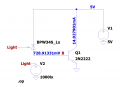#### LvW

Joined Jun 13, 2013
1,622
I don't need any references. I use a concept that allows me to simply calculate circuits and use reference data. Instead you suggest I use exponential functions.
No - I don´t. For determining the gain I only need to select the quiescent current Ic and with it the transconductance gm=Ic/Vt. Then, the resistors Rc and Re are selected and - as the the LAST step - I design the bias resistors, of course taking the voltage Vbe (assumed) and the base current into consideration. However, in some cases we even can neglect the base current when we have sufficient feedback (Barrie Gilbert says the base current is a "defect" and a "nuisance").

There is always a parasitic base resistance in a transistor and therefore no voltage is directly applied to the p-n junction. When a fast step voltage is applied to the base-emitter junction, the collector current does not appear immediately. It takes a current to appear, and then the carrier current causes carriers to be injected into the collector.
Why do you think that the existence of a parasitic base resistance will have any influence on the transistors working principle?
Are you aware what we are really doing while designing a simple resistive voltage divider? Only for calculation purposes we ASSUME that the total current would "produce" a part of the total applied voltage across each resistor. However, in reality, it is of course the voltage which appears across the resistor and allows a current. Without such a voltage there will be no E-field driving the current.

On the subject of there being no current sources.

Is a photodiode a current or voltage source?

Answer: Do you realize that we put 8 photocells IN SERIES (each app. 0.5 volts) to get 4 volts in total?

What controls the transistor in the optocoupler?

Answer: Same principle (photoeffect produces a voltage)

How is the transistor gain determined?

Answer: Gain (common emitter) is G=- gm*Rc/(1+gm*Re) with gm: Slope of the voltage-control characteristiv Ic=f(Vbe).

In a common base circuit, the gain is equal to the product of the injection coefficient and the charge carrier transfer coefficient. Both parameters characterize the charge carrier transfer. There are equations for the transistor using the notions of charge carriers in its regions.

Answer: The gain of a common base circuit is G=+gm*Rc (with gm as above).

Question: Where is any evidence for a current source?

#### Bordodynov

Joined May 20, 2015
3,037
In some strange way I see the answer.
From these answers I see that the answerer does not know the equivalent circuit of the photodiode (and photocell). The equivalent circuit is a current source equal to the photocurrent and a pn junction connected in parallel with it. Usually the photodiode is negatively biased and the reverse biased junction has almost no effect on the current. In this mode the photodiode is the current source. If the photodiode is not biased by negative voltage, the photocurrent will bias the pn junction and it will have 0.4 - 0.6 V.
The photo effect produces a photocurrent almost proportional to the light power and in no way is it a voltage source. Look at the large number of calculations of photodetectors. They draw an equivalent circuit as a current generator with a parallel capacitance (actually it is the capacitance of a pn junction).
Actually, I showed with my calculations the dependence of the current amplification by the transistor. The reference books show exactly this dependence. Apparently the one who wrote the answer did not understand what I showed.
By the way, the slope is proportional to the current. From the voltage the slope of the transistor depends on a "very convenient" exponential law.
Here is an example of a simple bipolar transistor amplifier circuit. With the device I measured the current amplification factor of the transistor. I wanted to set the current of the transistor to 1mA. Dividing this current by the obtained coefficient I got the base current and using Ohm's law I calculated the base current. Everything is simple. But try to calculate this circuit using the statement that the transistor is controlled by voltage. I don't think you can do it. That was the first circuit I soldered. I knew Ohm's law and how the current gain of the transistor is determined. I didn't know about the exponential dependence at that time and I didn't need it.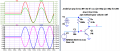Last edited:

#### LvW

Joined Jun 13, 2013
1,622
In some strange way I see the answer.
From these answers I see that the answerer does not know the equivalent circuit of the photodiode (and photocell). The equivalent circuit is a current source equal to the photocurrent and a pn junction connected in parallel with it. Usually the photodiode is negatively biased and the reverse biased junction has almost no effect on the current. In this mode the photodiode is the current source. If the photodiode is not biased by negative voltage, the photocurrent will bias the pn junction and it will have 0.4 - 0.6 V.
The photo effect produces a photocurrent almost proportional to the light power and in no way is it a voltage source. Look at the large number of calculations of photodetectors. They draw an equivalent circuit as a current generator with a parallel capacitance (actually it is the capacitance of a pn junction).
Bordodynov, I did not speak about "equivalent circuits". As you know, you can convert - on the paper !! - each voltage source into a current source and vice versa. So - such an equivalent diagram cannot explain the physics behind it.
As far as I have understood our discussion, we were discussing "physical effects" and real working principles. Of course, the photodiode - as each voltage source - can deliver a current when there is a closed loop. Who will deny this? But this current needs a driving force (like each current!). And this is the photo-voltage !
Therefore, I have mentioned the fact that it is common practice to put several photocells in series (would you do this with current sources?) for producing a higher voltage.
Here is an example of a simple bipolar transistor amplifier circuit. With the device I measured the current amplification factor of the transistor. I wanted to set the current of the transistor to 1mA. Dividing this current by the obtained coefficient I got the base current and using Ohm's law I calculated the base current. Everything is simple. But try to calculate this circuit using the statement that the transistor is controlled by voltage. I don't think you can do it. That was the first circuit I soldered. I knew Ohm's law and how the current gain of the transistor is determined. I didn't know about the exponential dependence at that time and I didn't need it.
Of course, I can do it.
More than that, using the voltage control feature is the only method you have used (perhaps without knowing).
Let me explain: In your calculation, did you use a value of app. 0.6...0.7V for Vbe? Of course, you did. Why?
Because you need such a voltage for "open" the BJT (allow a collector current).
This voltage was the start (first step) of your design.
As a second step, you have designed the bias circuitry which can produce such a voltage.
As you know, your example circuit is a very bad one and I am curious how close you were to the wanted current of 1mA.
Nevertheless, what you did was nothing else than to build a voltage divider between the base resistor and the base-emitter path. And for producing a desired voltage division you must know the current (Ib) through this division chain.
So you have back calculated Ib=Ic/B.
That means: You have designed a voltage divider that is able to produce the base-emitter voltage, which is necessary to allow the collector current. And, of course, it was necessary to know and to consider the current through the single bias resistor.
But this approach does not prove any current-control mechanism.

As you know, the classical (and best) approach for such a common emitter stage is to use a low-impedance voltage divider at the base for producing a voltage Vb as "stiff" as possible (as independent as possible from the unknown base current) - together with an emitter resistor Re for producing negative feedback.
In this case, the whole circuit is relatively independent on the above mentioned Vbe-uncertainty (0.6 or 0.7 volts) as well as the huge tolerances of Ib.
Why do you think is this the best design?
Are you aware that the effect of Re is current-controlled voltage feedback?

Do you really consider my position still as "strange"?

Final question: In your design example, it was not necessary to know about the exponential current-voltage relationship because you did not want to have a specific voltage gain. So - you have selected 1mA without any performance requirement. However, as soon as you want to design a gain stage with specific properties you need to know the transconductance, don`t you?

Last edited:

#### Bordodynov

Joined May 20, 2015
3,037
I have a lot of experience with circuit calculations. When I was young, I soldered many direct amplifier receivers, which I designed myself. I also did active antennas and antenna amplifiers. And I'm a former integrated circuit designer. I've been doing that for 20 years. I'm also an expert at modeling with Spice. Just so you know who you are talking to. Right now I'm in the business of turning lasers on. I design photodetectors. And I never used the "crappy" basic divider and resistor circuit, which is a poor use of transistors and is basic for students to learn.
Let's get back to our sheep.
First I will show that you don't know how a photodiode works. I will write another letter about the calculation of my first amplifier.
Photodiodes are usually used at negative bias. Their current is almost independent of this bias, but the higher the bias, the lower the barrier capacitance, which is needed to increase the speed. How do you explain from your position the independence of current from voltage change? Here is a calculation on LTspice. Although I don't think this is an argument for you. It is true that avalanche photodiodes have carrier multiplication at quite high voltages. By the way, I made models for them. Here you can see the dependence of the photocurrent on the voltage on the photodiode.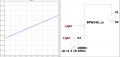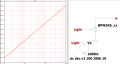Last edited:

#### Bordodynov

Joined May 20, 2015
3,037
Let's get back to my amplifier. That was the time of germanium transistors and your statement about "Vbe-uncertainty (0.6 or 0.7 volts)" is wrong. Look at my calculation. I gave the voltage at the base. I used a 9 volt battery. When I did the calculation I did not consider the base-emitter voltage (I ignored it). This gave an error of 2%. The resistors had a 5% variation. I wanted to set 1 mA through the transistor and I got this current (I used a cheap ammeter with an arrow). I was making a circuit on a particular transistor and I had no trouble making a current gain measurement for that particular transistor. Earlier, I had bought an amateur radio kit. This kit included a meter, a transistor meter attachment, and a soldering iron. I am well aware that if I put a transistor with a different current gain into the circuit, I would have to change the basic mode.
The mathematical model of the Ebers-Moll transistor uses current transfer coefficients, although there are also diodes, with exponential volt-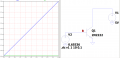ampere characteristics. It turns out more important in the model currents through diodes. In very rare cases voltages are used to set the mode of the transistor. A small change in the base-emitter voltage can significantly change the collector current.